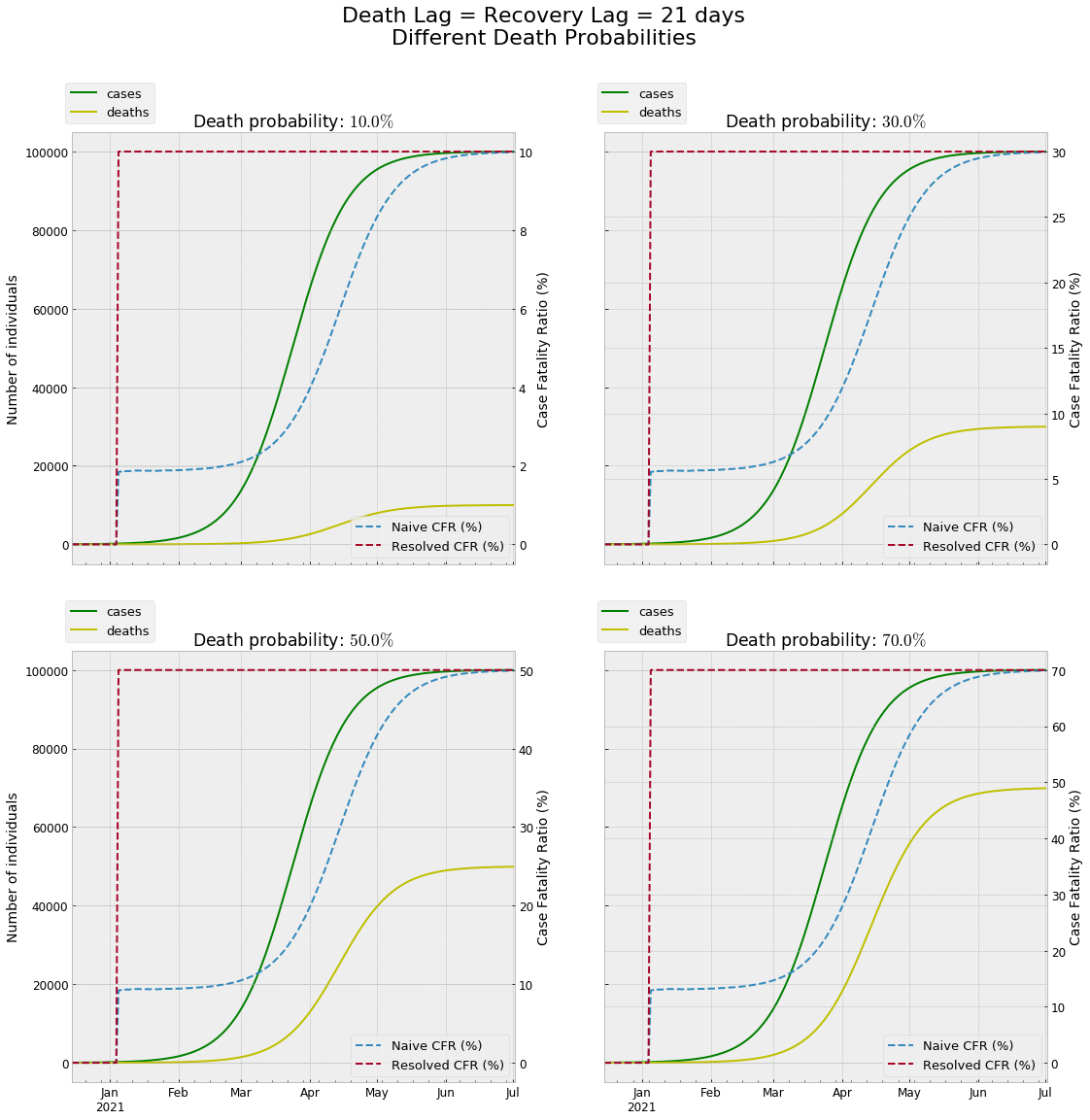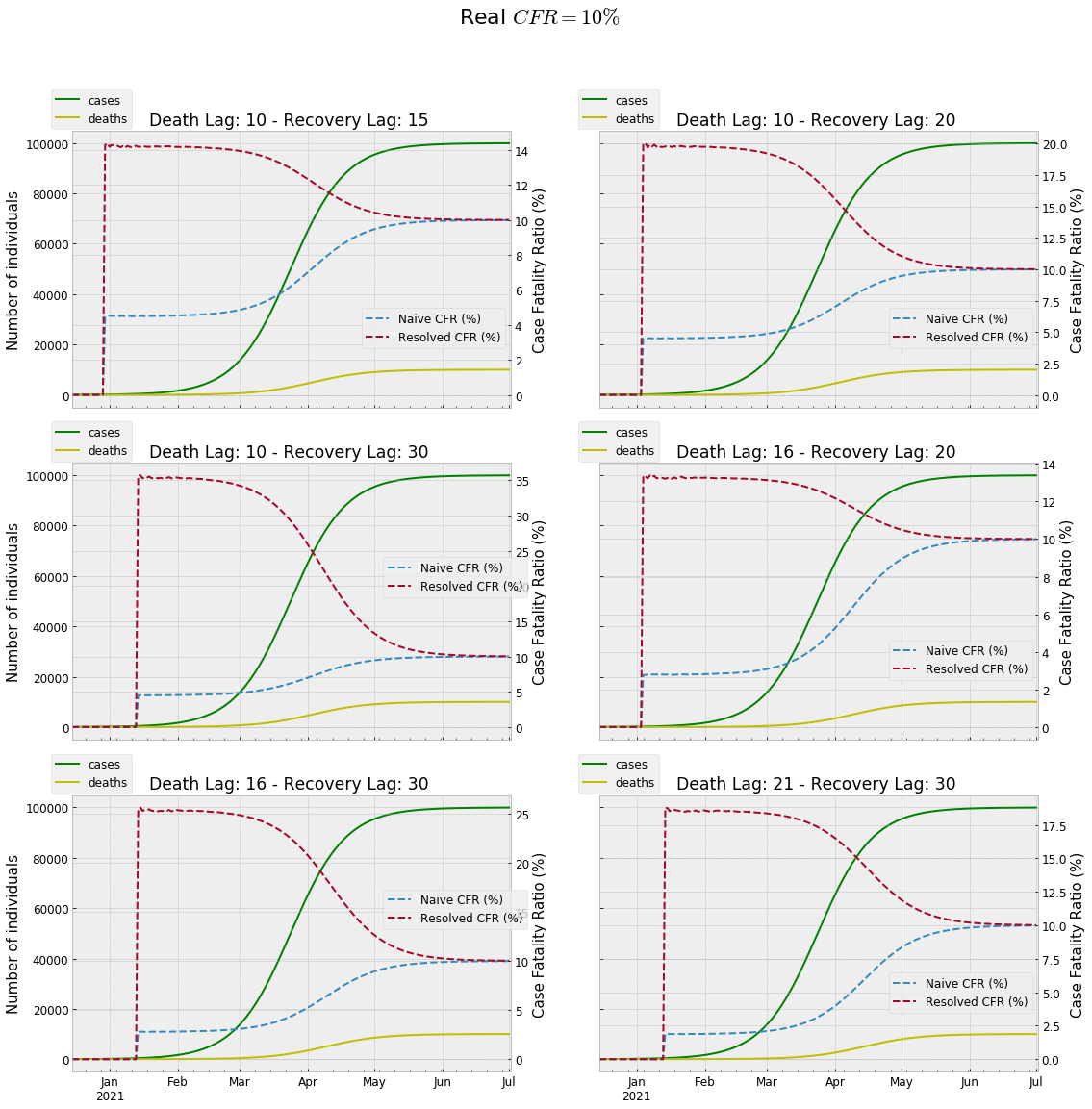In :
from IPython.display import display
from IPython.display import HTML
import IPython.core.display as di # Example: di.display_html('<h3>%s:</h3>' % str, raw=True)

# This line will hide code by default when the notebook is exported as HTML
di.display_html('<script>jQuery(function() {if (jQuery("body.notebook_app").length == 0) { jQuery(".input_area").toggle(); jQuery(".prompt").toggle();}});</script>', raw=True)

# This line will add a button to toggle visibility of code blocks, for use with the HTML export version
di.display_html('''<button onclick="jQuery('.input_area').toggle(); jQuery('.prompt').toggle();">Toggle code</button>''', raw=True)

In :
import numpy as np, pandas as pd, matplotlib.pyplot as plt
plt.style.use('bmh')
import math
import matplotlib
matplotlib.rcParams.update({'font.size': 12, 'font.family': 'sans-serif'})
pd.options.display.float_format = '{:.3f}'.format


# Some considerations about the Case Fatality Ratio of Covid-19

This notebook study was born by the interest aroused by reading the following article about the Case Fatality Ratio (CFR) of Covid19: Mark Bevand Blog
I highly suggest to read it before to dive into this notebook.

Let's focus on two ways to estimate the case fatality ratio (CFR) :

Naive Estimator = Current Deaths / Current Cases
Resolved Estimator = Current Deaths / (Current Deaths + Current Recovered)

The aim of this notebook is not to compute the unbiased estimate of the CFR, because in order to do that we have to model other factors and make other assumptions (check out the final part of the Mark Bevand Blog).
The aim is to understand if and why we should prefer the Naive Estimator or the Resolved Estimator in order to infer the severity of the epidemic.

We import the Covid-19 data of the most infected Countries at 28th February 2020.

In :
data = pd.read_excel('D:\Covid19\data_28feb.xlsx').set_index('Country')


In order to compute a weighted average we calculate the countries weighs by adding a new column.

In :
data['weigh'] = data['Cases'] / data['Cases'].sum(axis = 0)
data

Out:
Cases Deaths Recovered Critical weigh
Country
China 78832 2788 36456 7952 0.941
S.Korea 2337 16 24 7 0.028
Italy 888 21 46 64 0.011
Diamond Princess 705 6 10 34 0.008
Iran 388 34 73 0 0.005
Japan 226 5 32 13 0.003
Singapore 96 0 69 8 0.001
Hong Kong 93 2 18 6 0.001
Usa 62 0 6 1 0.001
Germany 60 0 16 2 0.001
France 57 2 11 1 0.001

Let's calculate the two current estimates:

In :
naive_est = (data['Deaths'] / data['Cases'] * data['weigh']).sum()
resolved_est = (data['Deaths'] / (data['Deaths']+data['Recovered']) * data['weigh']).sum()

In :
cfr = pd.DataFrame(np.array([naive_est, resolved_est])*100, columns = ['CFR'], \
index = ['Naive Estimator (%)', 'Resolved Estimator (%)'])
cfr

Out:
CFR
Naive Estimator (%) 3.432
Resolved Estimator (%) 8.657

We know that at the end of the pandemic the two metrics must coincide.
But in the middle of the pandemic they would tipically differ.

The problem is: which of the two estimators is more biased during the pandemic?

It seems that the Naive Estimator underestimates the real value, because the pandemic is still ongoing.
On the contrary the Resolved Estimator seems to overestimate the real value.

We are going to show that if we use a logistic growth model for the epidemic (slow increasing initially, rapid exponentially increasing after a while and negative 2nd derivative after the temporal mid point of the pandemic (when quarantines start working) ), the bias of the two measures doesn't depend on the death probability , but only on the death lag (the average number of days between diagnosis and death) and the recovery lag (the average number of days between diagnosis and recovery).

We are going to highlight that if those lags coincide hence the Resolved Estimator computes a perfect estimate of the real value, at any day.

Let's define a new variable, the Recovery multiple.
Recovery multiple = Recovery Lag / Death Lag

This notebook will highlight the following results:

If the Recovery Multiple is $< 1.5$ the Naive CFR bias is higher than the Resolved Estimator bias.
In other words: Naive CFR underestimates too much the real value compared to how Resolved Estimator overestimates.

If the Recovery Multiple is $\approx 1.5$ they will have a similar bias (Naive CFR would underestimate in the same manner as Resolved CFR would overestimate).

If the Recovery Multiple is $>= 2$ the Naive CFR bias is smaller than the Resolved Estimator bias.
In other words: Resolved Estimator overestimates too much the real CFR compared to how Naive Estimator underestimates.

In our simulation we assume that pandemic will last 200 days.
The accuracy of this assumption is not fundamental for our aim.
We use a growth rate of the logistic function $k = 0.08$, as the author of original article did.

Let's start by showing that, regardless the death probability $p$, if the death lag is equal to the recovery lag, the Naive CFR ratio always underestimates the true CFR, while the Resolved CFR is a perfect estimator.

In :
def sim(p = 0.1, time_to_death = 21, time_to_heal = 21, k = 0.08):
population = 100_000
days = 200
death_prob = p
time_to_death = time_to_death
time_to_heal = time_to_heal
hist =  # no cases before the storm
deaths = recovs = naive_cfr = resolved_cfr = 0
df = pd.DataFrame(index = pd.date_range(start = '15-12-2020', periods = days), \
columns = 'day,cases,deaths,recoveries,Naive CFR (%),Resolved CFR (%)'.split(','))

for d in range(1, days):
cases = round(population / (1 + math.e**(-k*(d - days/2))))
hist.append(cases)

if d >= time_to_death + 1:
new_cases_lagged = hist[d - time_to_death] - hist[d - time_to_death - 1]
new_deaths_lagged = (new_cases_lagged) * death_prob
deaths += new_deaths_lagged

if d >= time_to_heal + 1:
new_cases_lagged = hist[d - time_to_heal] - hist[d - time_to_heal - 1]
new_recovs_lagged = (new_cases_lagged) * (1 - death_prob)
recovs += new_recovs_lagged

if d > max(time_to_death, time_to_heal):
if cases:
naive_cfr = 100 * deaths / cases
if deaths + recovs:
resolved_cfr = 100 * deaths / (deaths + recovs)

df.iloc[d-1] = [d, cases, deaths, recovs, naive_cfr, resolved_cfr]
return df

In :
%%javascript
IPython.OutputArea.prototype._should_scroll = function(lines) {
return false;
}

In :
fig, ax = plt.subplots(2,2, sharex=True, sharey=True, figsize = (18,18))
fig.suptitle(f'Death Lag = Recovery Lag = 21 days\nDifferent Death Probabilities', fontsize = 22)
death_probs = [0.1, 0.3, 0.5, 0.7]
j = 0
for i in range(0,2):
for k in range(0,2):
df = sim(p = death_probs[j])
ax1 = ax[i,k].twinx()
df[['Naive CFR (%)', 'Resolved CFR (%)']].plot(linestyle = '--', ax = ax1);
df[['cases','deaths']].plot(ax = ax[i,k], label = ['Cum. Cases', 'Cum. Deaths'], color = ['g','y']);
ax[i,k].set_title(f'Death probability: ${death_probs[j]*100}\%$')
ax[i,k].set_ylabel('Number of individuals', fontsize = 14)
ax1.set_ylabel('Case Fatality Ratio (%)', fontsize = 14)
ax[i,k].legend(bbox_to_anchor=(0, 0.93, 0.2, 0.2), fontsize = 13)
ax1.legend(loc = 4, fontsize = 13);
j += 1Now instead we want to hypothesize that:
death lag $\neq$ recovery lag , and we set the death probability $p = 0.1$.
Hence the real $CFR = 0.1$. We can choose every $p \in (0,1)$. The choice of $p$ doesn't alter the results.

In this case the two CFR metrics will differ.
We are going to use 6 combinations of lags, where the first element of each tuple is the death lag and the second element of the same tuple is the recovery lag.
We have realistically chosen a recovery lag always higher than death lag.

In :
from itertools import product

In :
lag_death = [10,16,21] # list of days before death
lag_recover = [15,20,30] # list of days before recovery

In :
combos = product(lag_death, lag_recover)

# we want realistically consider only combos in which recovery takes longer than death
combos = [combo for combo in list(combos) if combo < combo ]
combos

Out:
[(10, 15), (10, 20), (10, 30), (16, 20), (16, 30), (21, 30)]

By fixing different lags for death and recovery, with recovery lag $>$ death lag , we find that:

1) If the ratio recovery lag / death lag $<$ 1.5 the Naive CFR underestimation bias is larger than the Resolved CFR overestimation bias. (Fig. (2x2) )

1) If the ratio recovery lag / death lag $\approx$ 1.5 the Naive CFR underestimation has the same bias compared to the Resolved CFR overestimation. (Fig. (1x1))

2) If the ratio recovery lag / death lag $>=$ 2 the Naive CFR underestimation bias is minor than the Resolved CFR overestimation bias. (Fig. (2x1) and (1x2))

In :
fig, ax = plt.subplots(3,2, sharex=True, sharey=True, figsize = (18,18))
fig.suptitle('Real $CFR = 10\%$', fontsize = 22)
pivot = 0
for i in range(0,3):
for k in range(0,2):
df = sim(time_to_death = combos[pivot], time_to_heal = combos[pivot])
ax1 = ax[i,k].twinx()
df[['Naive CFR (%)', 'Resolved CFR (%)']].plot(linestyle = '--', ax = ax1);
df[['cases','deaths']].plot(ax = ax[i,k], label = ['Cum. Cases', 'Cum. Deaths'], color = ['g','y']);
ax[i,k].set_title(f'Death Lag: {combos[pivot]} - Recovery Lag: {combos[pivot]} ')
ax[i,k].set_ylabel('Number of individuals', fontsize = 15)
ax1.set_ylabel('Case Fatality Ratio (%)', fontsize = 15)
if (i == 1 and k == 0) or (i == 2 and k == 0):
ax1.legend(bbox_to_anchor=(0.85, 0.33, 0.2, 0.35), fontsize = 12)
else:
ax1.legend(bbox_to_anchor=(0.9, 0.03, 0.1, 0.35), fontsize = 12);
ax[i,k].legend(bbox_to_anchor=(-0.05, 0.97, 0.2, 0.20), fontsize = 12)
pivot += 1So the crucial final question is:

Which are the average death lag and the average recovery lag of this epidemic to use in these simulations?

At this point we turn the same question over epidemiologists and virologists, who can surely know better than us the answer.